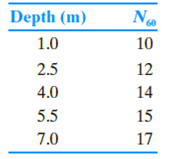Chapter 17, Problem 17.3PPrinciples of Geotechnical Enginee...

9th Edition
Braja M. Das + 1 other
ISBN: 9781305970939

Solutions

Chapter
SectionPrinciples of Geotechnical Enginee...

9th Edition
Braja M. Das + 1 other
ISBN: 9781305970939
Textbook Problem

The following are the results of a standard penetration test in sand. Determine the corrected standard penetration numbers, (N1)60, at the various depths given. Note that the water table was not found during the boring operation. Assume that the average unit weight of sand is 18.7 kN/m3. Use Liao and Whitman’s relationship [Eq. (17.10)]. Assume pa ≈ 100 kN/m2.To determine

Find the corrected standard penetration numbers (N1)60 at the various depth.

Explanation

Given information:

Show the depth and number of blows shown in table:

 Depth,(m) N60 1 10 2.5 12 4 14 5.5 15 7 17

Assume the average unit weight (γ) of sand is 18.7kN/m3.

The atmospheric pressure is (pa) 100kN/m2.

Calculation:

Calculate the overburden pressure (σo) at depth 1m using the relation:

(σo)=γ×depth (1)

Substitute 1m for depth and 18.7kN/m3 for (γ) in Equation (1).

(σo)=18.7×1=18.7kN/m2

Find the correction factor (CN) at depth 1m using Liao and Whiteman’s relation:

CN=[1(σopa)]0.5 (2)

Here, (σo) is overburden pressure.

Substitute 18.7kN/m2 for σo and 100kN/m2 for pa in Equation (2).

CN=[1(18.7100)]0.5=[5.3475]0.5=2.31

Find the corrected standard penetration numbers (N1)60 at 1m depth.

(N1)60=CNN60 (3)

Here, (CN) is correction factor and N60 is standard penetration number.

Substitute 2.31 for CN and 10 for N60 in Equation (3).

(N1)60=2.31×10=23.123

Thus, the corrected standard penetration numbers at the depth 1m is 23.

Calculate the overburden pressure (σo) at depth 2.5m using the relation:

Substitute 2.5m for depth and 18.7kN/m3 for (γ) in Equation (1).

(σo)=18.7×2.5=46.75kN/m2

Find the correction factor (CN) at depth 2.5m using Liao and Whiteman’s relation:

Substitute 46.75kN/m2 for σo and 100kN/m2 for pa in Equation (2).

CN=[1(46.75100)]0.5=[2.1390]0.5=1.462

Find the corrected standard penetration numbers (N1)60 at 2.5m depth.

Substitute 1.462 for CN and 12 for N60 in Equation (3).

(N1)60=1.462×12=17.5418

Thus, the corrected standard penetration numbers at the depth 2.5m is 18.

Calculate the overburden pressure (σo) at depth 4m using the relation:

Substitute 4m for depth and 18.7kN/m3 for (γ) in Equation (1)

Still sussing out bartleby?

Check out a sample textbook solution.

See a sample solution

The Solution to Your Study Problems

Bartleby provides explanations to thousands of textbook problems written by our experts, many with advanced degrees!

Get Started

Solve Problem 20.21 using Excel.

Engineering Fundamentals: An Introduction to Engineering (MindTap Course List)

Why is an object said to have greater semantic content than an entity?

Database Systems: Design, Implementation, & Management

What function does the compound rest serve?

Precision Machining Technology (MindTap Course List)

How can virtualization save an organization money?

Principles of Information Systems (MindTap Course List)

What is benchmarking?

Management Of Information Security

What are some examples of internal and external sources that a decision support system might use?

Enhanced Discovering Computers 2017 (Shelly Cashman Series) (MindTap Course List)

What arc problems can occur in deep or narrow weld joints?

Welding: Principles and Applications (MindTap Course List)